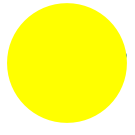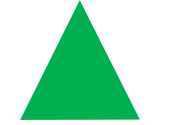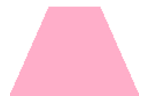Smartick is an advanced online program that teaches kids math and coding in only 15 min. a day

Dec23

# Geometric Plane Shapes: Circles, Triangles, Rectangles, Squares, and Trapezoids

What are geometric plane shapes? What characteristics do they have? These are the questions that we will answer in this post.

The principal geometric plane shapes are:

## The CircleThe circle is a shape that can be made by tracing a curve that is always the same distance from a point that we call the center. The distance around a circle is called the circumference of the circle.

## The TriangleThe triangle is a shape that is formed by 3 straight lines that are called sides. There are different ways of classifying triangles, according to their sides or angles.

1. According to their angles:
• Right triangle: the largest of the 3 angles is a right angle.
• Acute Triangle: the largest of the 3 angles is an acute angle (less than 90 degrees).
• Obtuse Triangle: the largest of the 3 angles is an obtuse angle (more than 90 degrees).

2. According to their sides:

• Equilateral Triangle: all 3 sides are the same length.
• Isosceles Triangle: it has 2 (or more) sides that are of equal length. (An equilateral triangle is also isosceles.)
• Scalene Triangle: no 2 sides are of equal measure.

## The RectangleThe rectangle is a shape that has 4 sides. The distinguishing characteristic of a rectangle is that all 4 angles measure 90 degrees.

## The RhombusThe rhombus is a shape formed by 4 straight lines. Its 4 sides measure the same length but, unlike the rectangle, any of all 4 angles measure 90 degrees.

## The SquareThe square is a type of rectangle, but also a type of rhombus. It has characteristics of both of these. That is to say, all 4 angles are right angles, and all 4 sides are equal in length.

## The TrapezoidThe trapezoid also has 4 sides. It has two sides that are parallel but the other 2 are not.

You can practice with the geometric plane shapes by registering in Smartick.

• Kwabena Nyamekye Adjepong.Jun 02 2020, 10:42 AM

Like the site.

• GloryJun 02 2020, 7:19 AM

Nice and easy to understand,my kids enjoyed it!!!

• Ugwu DestinyMay 25 2020, 5:23 AM

Thank you Mrs Mercy

• Ugwu DestinyMay 25 2020, 5:17 AM

Nice lesson,Thank you

• Kelly SimpsonApr 24 2020, 9:01 AM

Please can you put more shapes???!!!!!

• Abeer MizanApr 15 2020, 3:50 AM

thank you for the lesson!

• AlinaSep 18 2019, 3:38 PM

Thank the lord. I couldn’t tell the difference between square and trapezoid

• HerobrineMay 13 2019, 1:04 AM

Thank you for my lesson

• Yola BingaliMar 28 2019, 11:16 AM

Nice!

• Sai KumarDec 22 2018, 11:24 AM

2D square problem

• NewcomerJan 17 2019, 1:54 PM

There are other shapes worth considering.

• AmnaFeb 17 2018, 3:32 AM

I enjoyed learning different geometrical shapes

• SanikaOct 28 2017, 9:58 AM

You should tell more about each

• HemanthJul 17 2019, 9:34 AM

Nice